17 图像分类（上）：图像分类原理与图像分类模型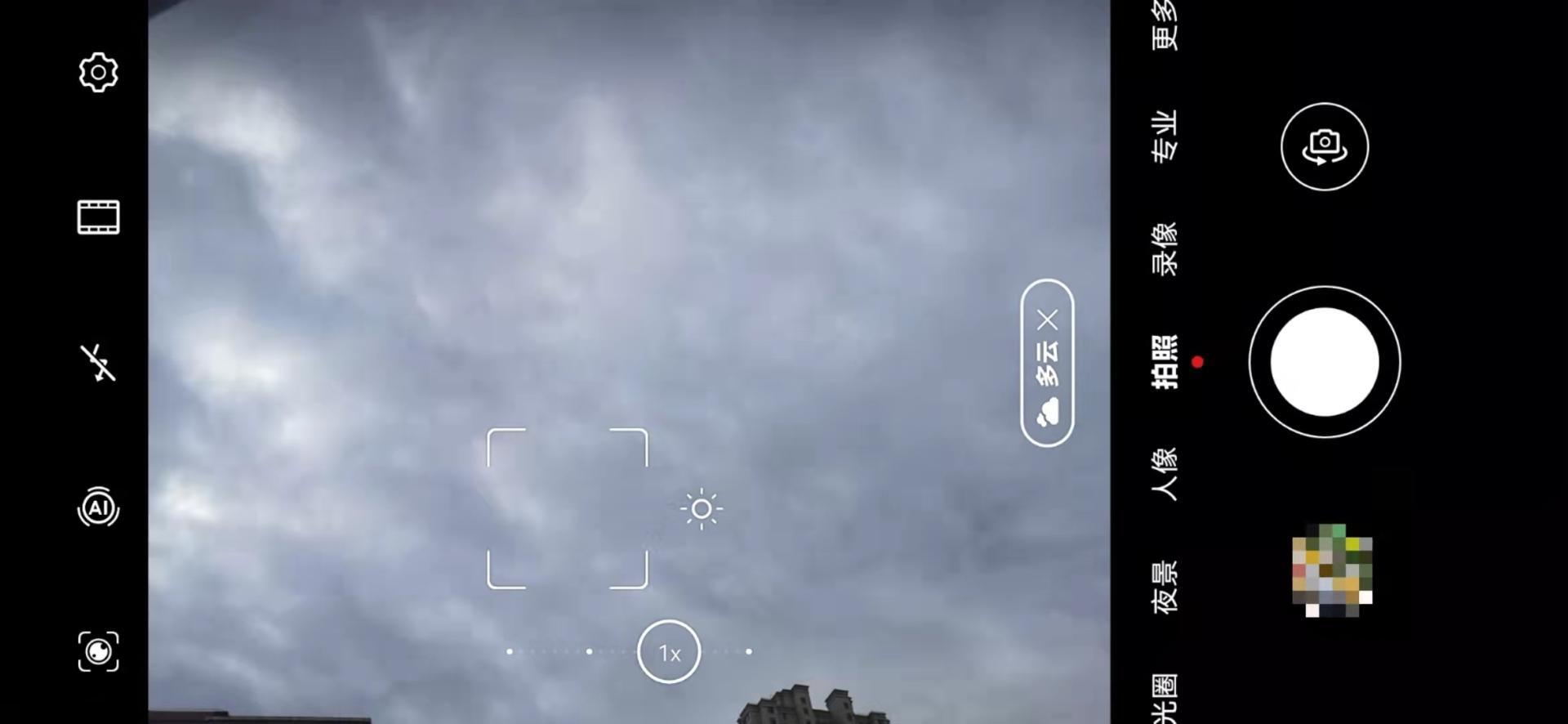## 图像分类原理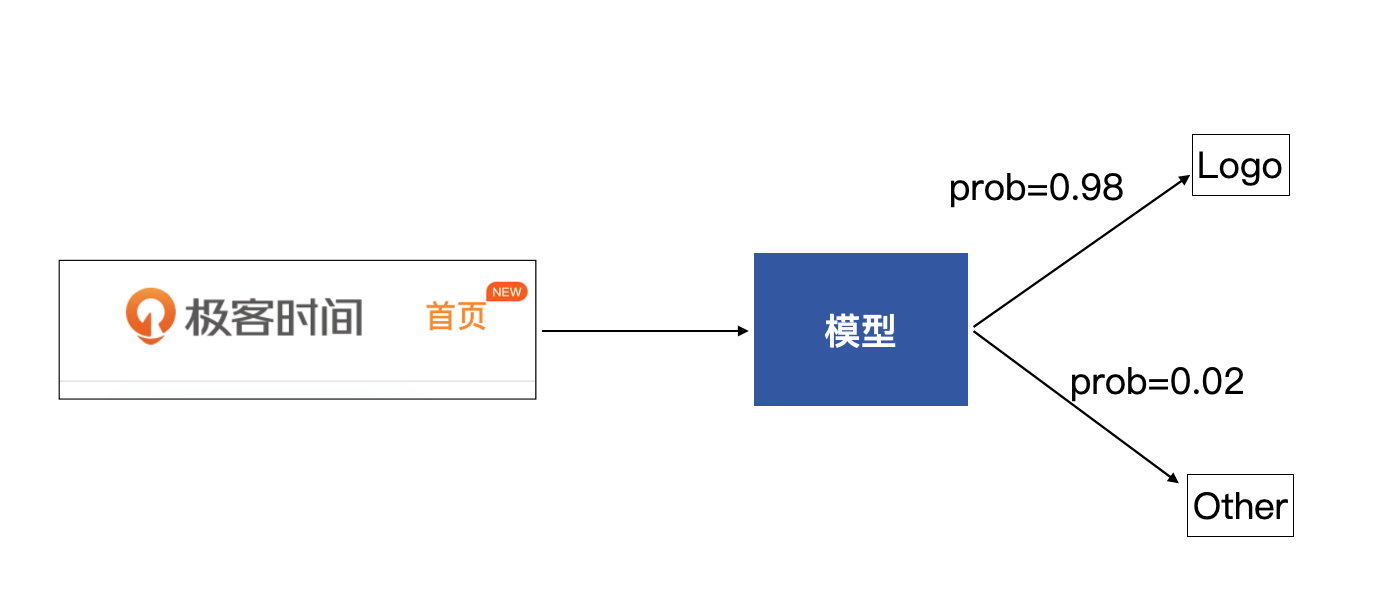### 感知机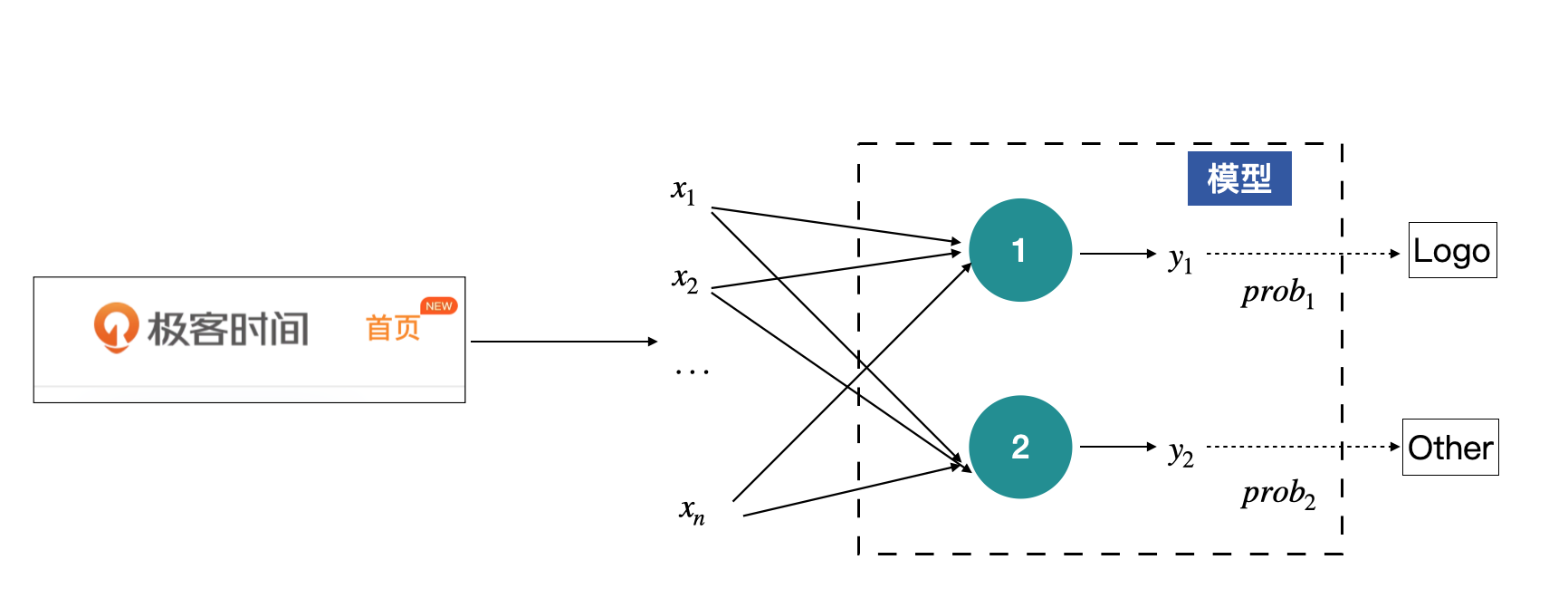$y\_i=\\delta(w\_{i1}x\_{1} + w\_{i2}x\_{2} + … + w\_{i\_n}x\_{n} + b\_i), \\space \\space \\space i=1,2$

Softmax的计算公式如下：

$\\delta(x\_j) = \\frac{e^{x\_j}}{\\sum\_{j=1}^{m}e^{x\_j}}$

import torch
import torch.nn as nn

# 2个神经元的输出y的数值为
y = torch.randn(2)
print(y)

m = nn.Softmax(dim=0)
out = m(y)
print(out)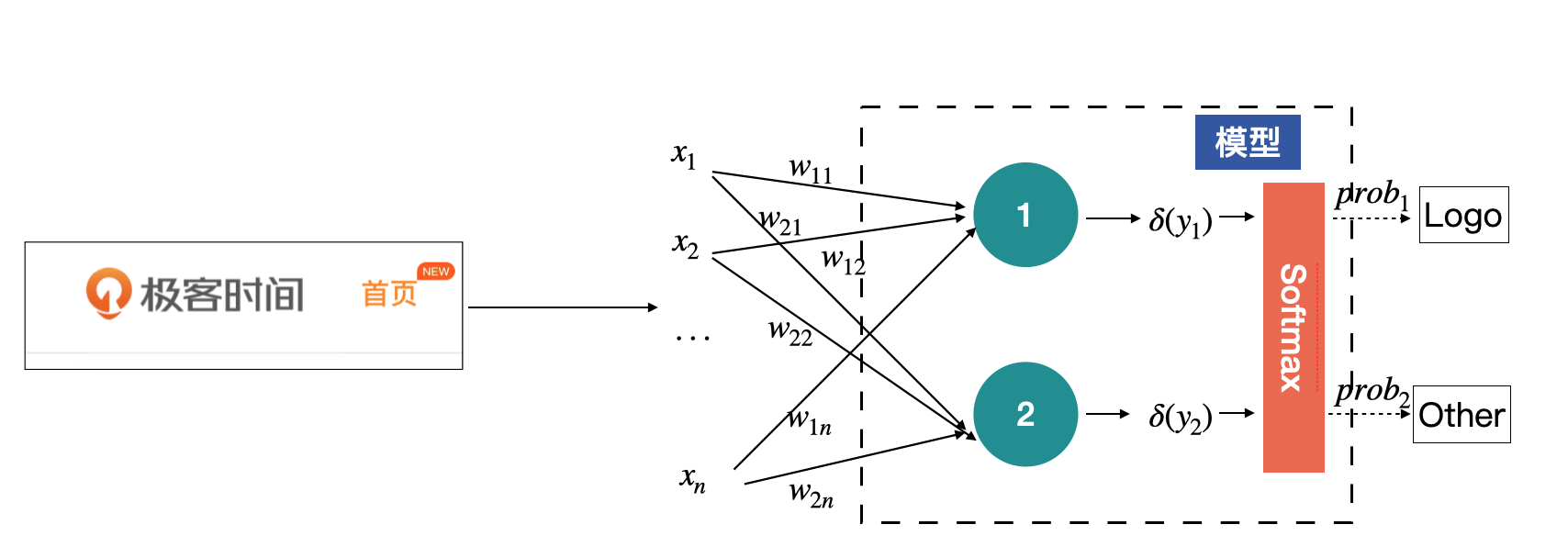### 全连接层

x = torch.randint(0, 255, (1, 128*128), dtype=torch.float32)
fc = nn.Linear(128*128, 2)
y = fc(x)
print(y)

# 注意y的shape是(1, 2)
output = nn.Softmax(dim=1)(y)
print(output)



• in_features：输入特征的个数，在本例中为128x128；
• out_features：输出的特征数，在本例中为2；
• bias：是否需要偏移项，默认为True。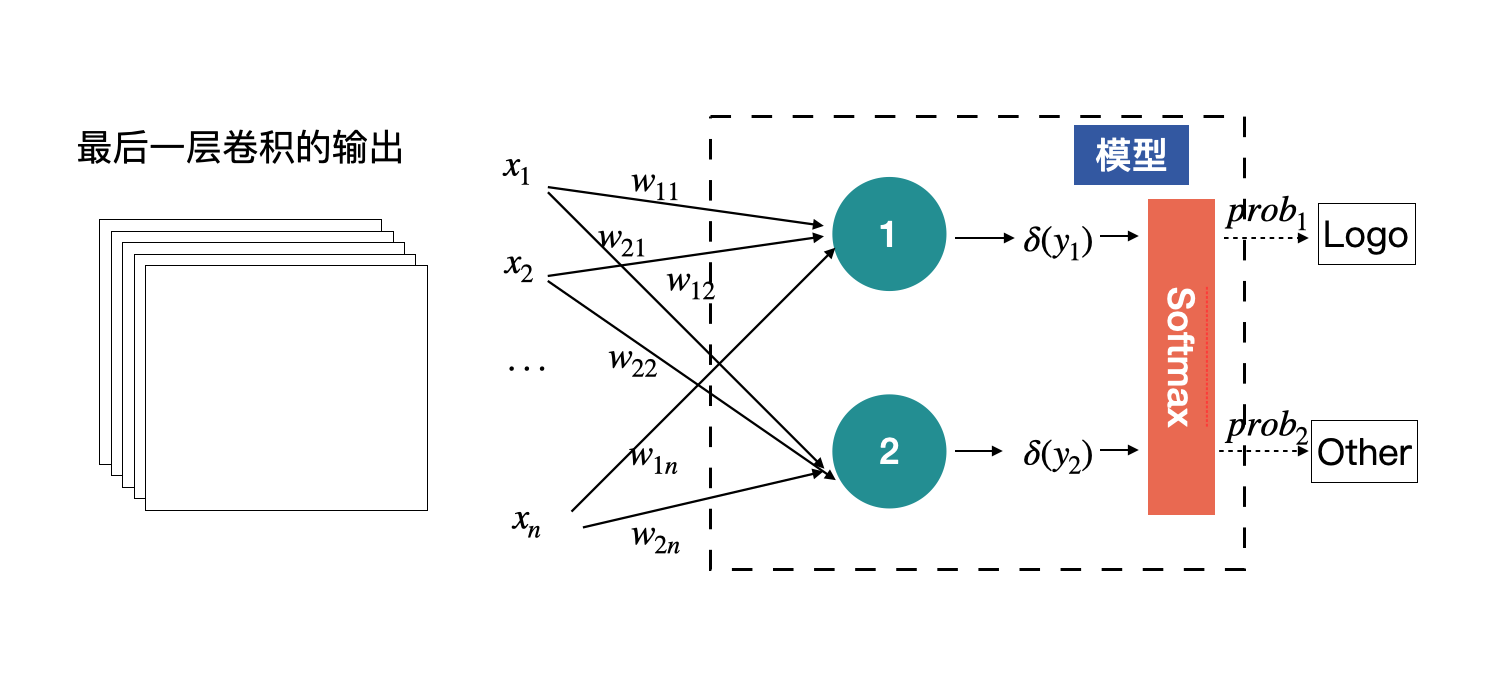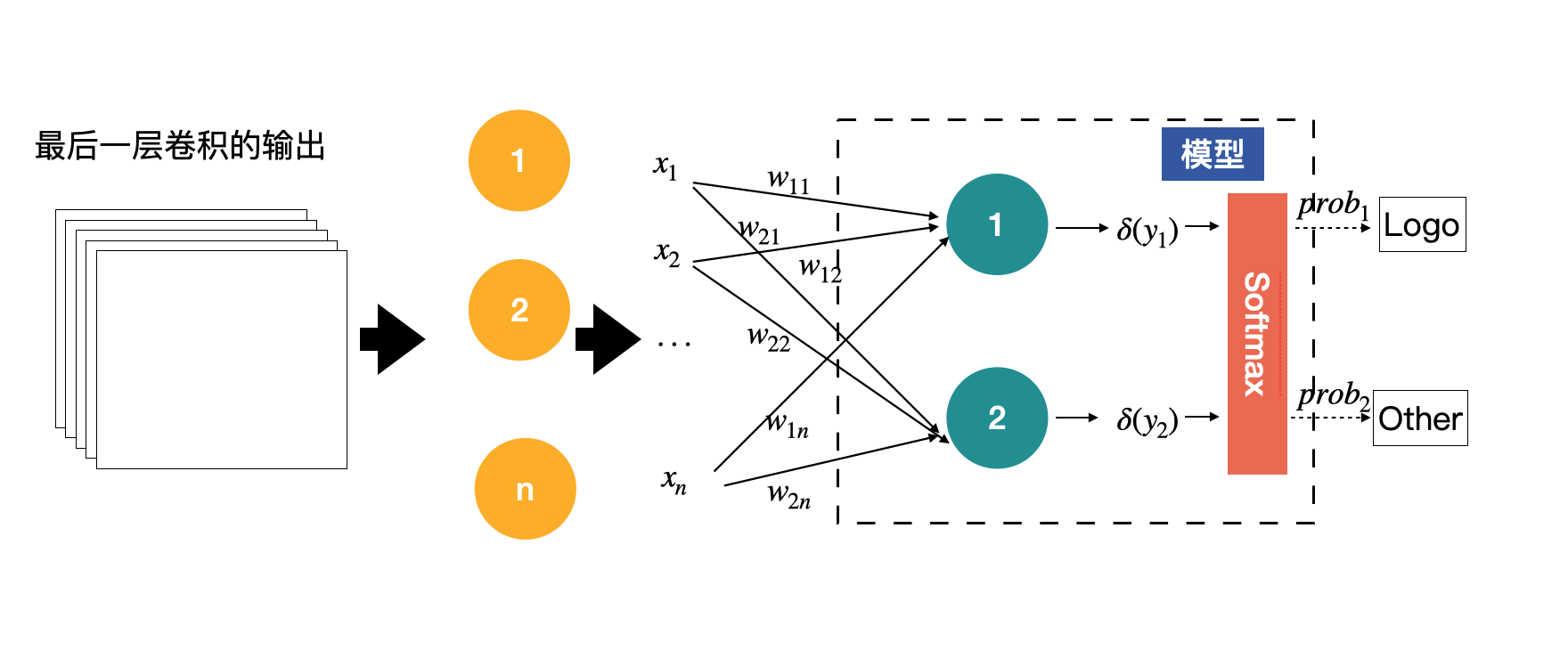## 卷积神经网络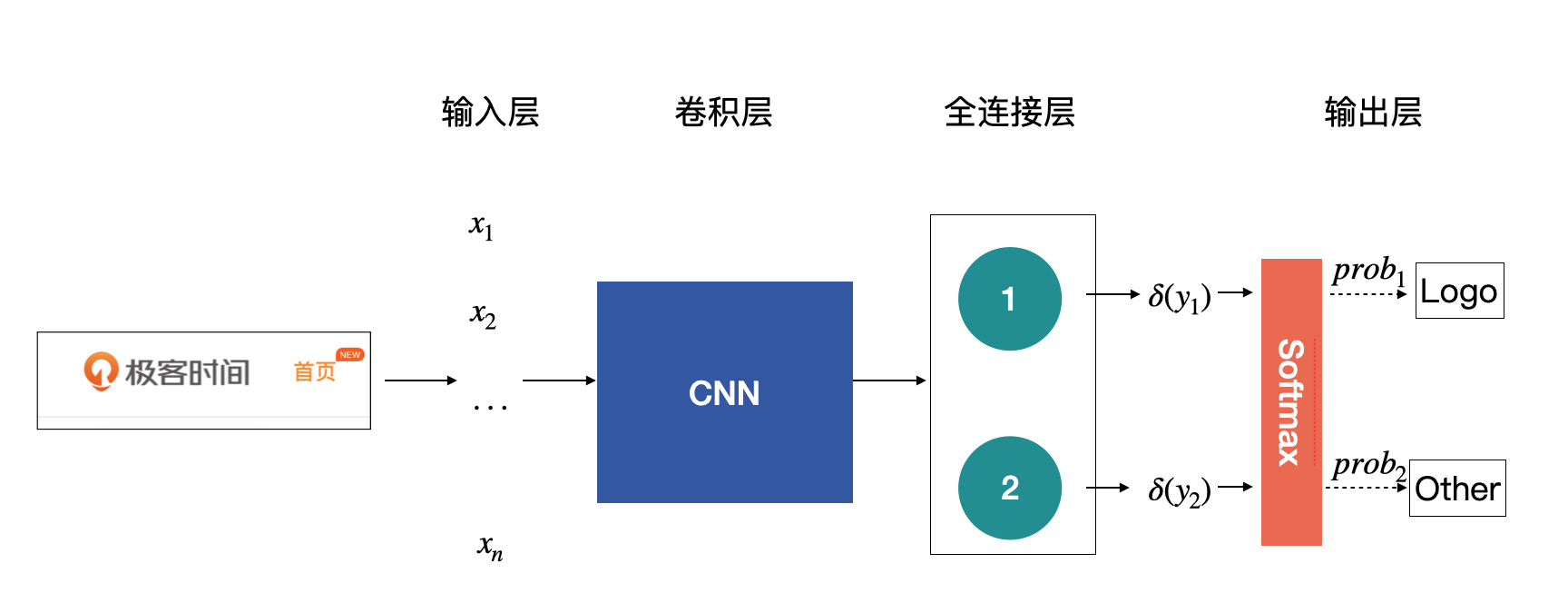### ImageNet

ImageNet本身包含了一个非常大的数据集，并且从2010年开始，每年都会举办一次著名的ImageNet 大规模视觉识别挑战赛（The ImageNet Large Scale Visual Recognition Challenge ，ILSVRC），比赛包含了图像分类、目标检测与图像分割等任务。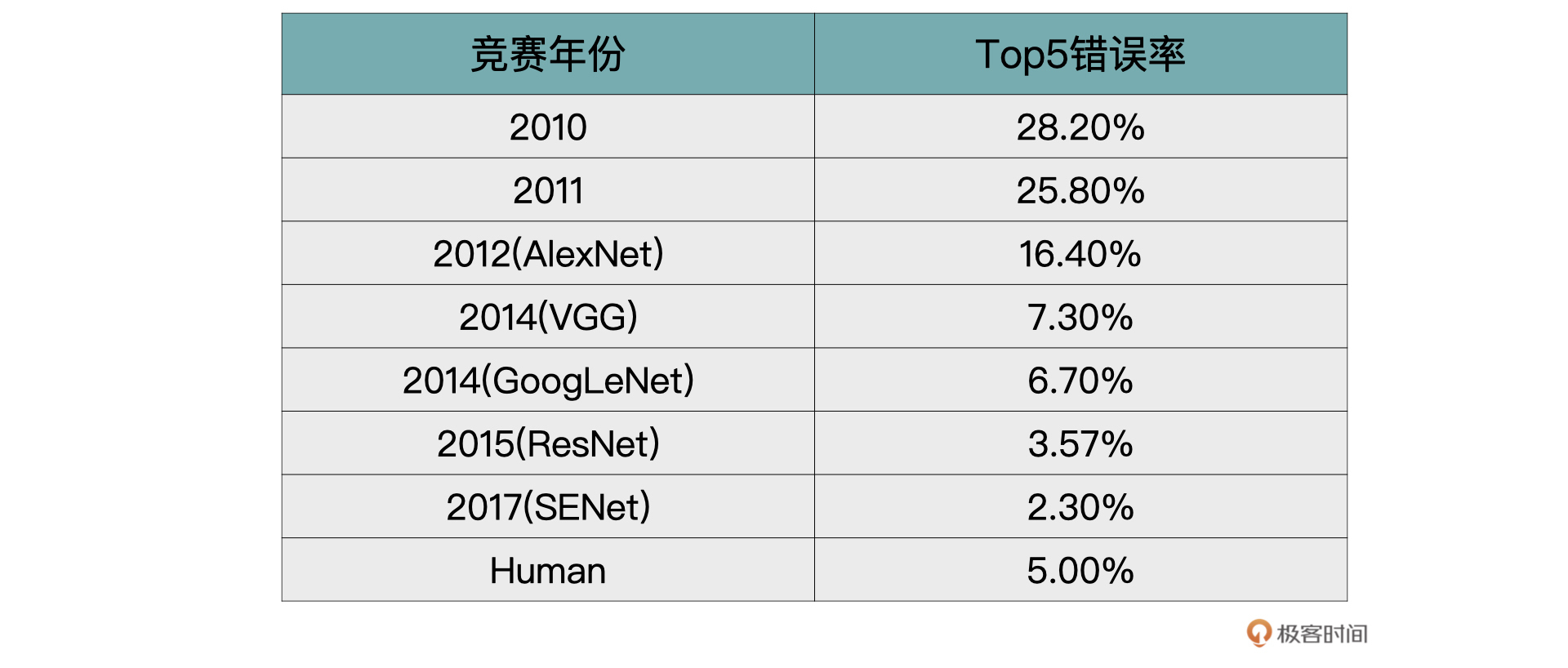### VGG

VGG取得了ILSVRC 2014比赛分类项目的第2名和定位项目的第1名的优异成绩。

1. 证明了随着模型深度的增加，模型效果也会越来越好。
2. 使用较小的3x3的卷积，代替了AlexNet中的11x11、7x7以及5x5的大卷积核。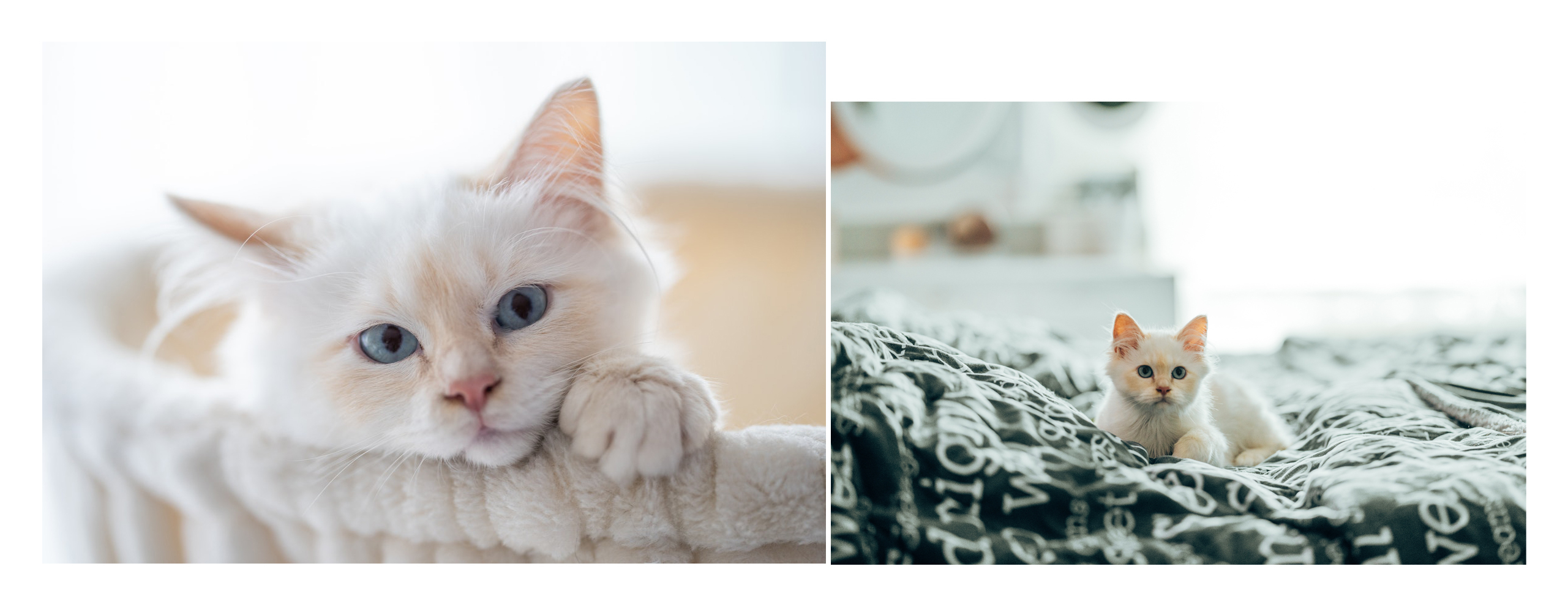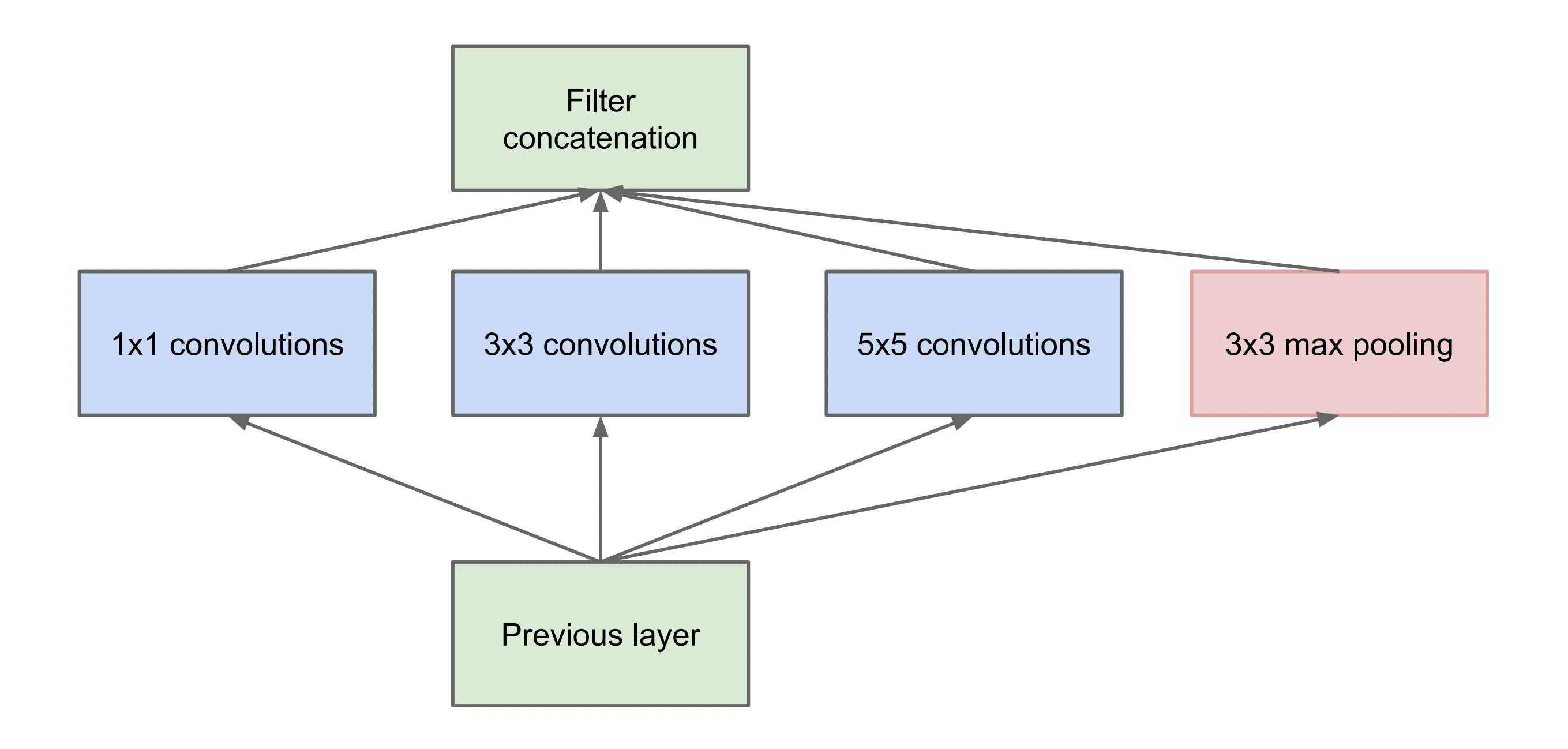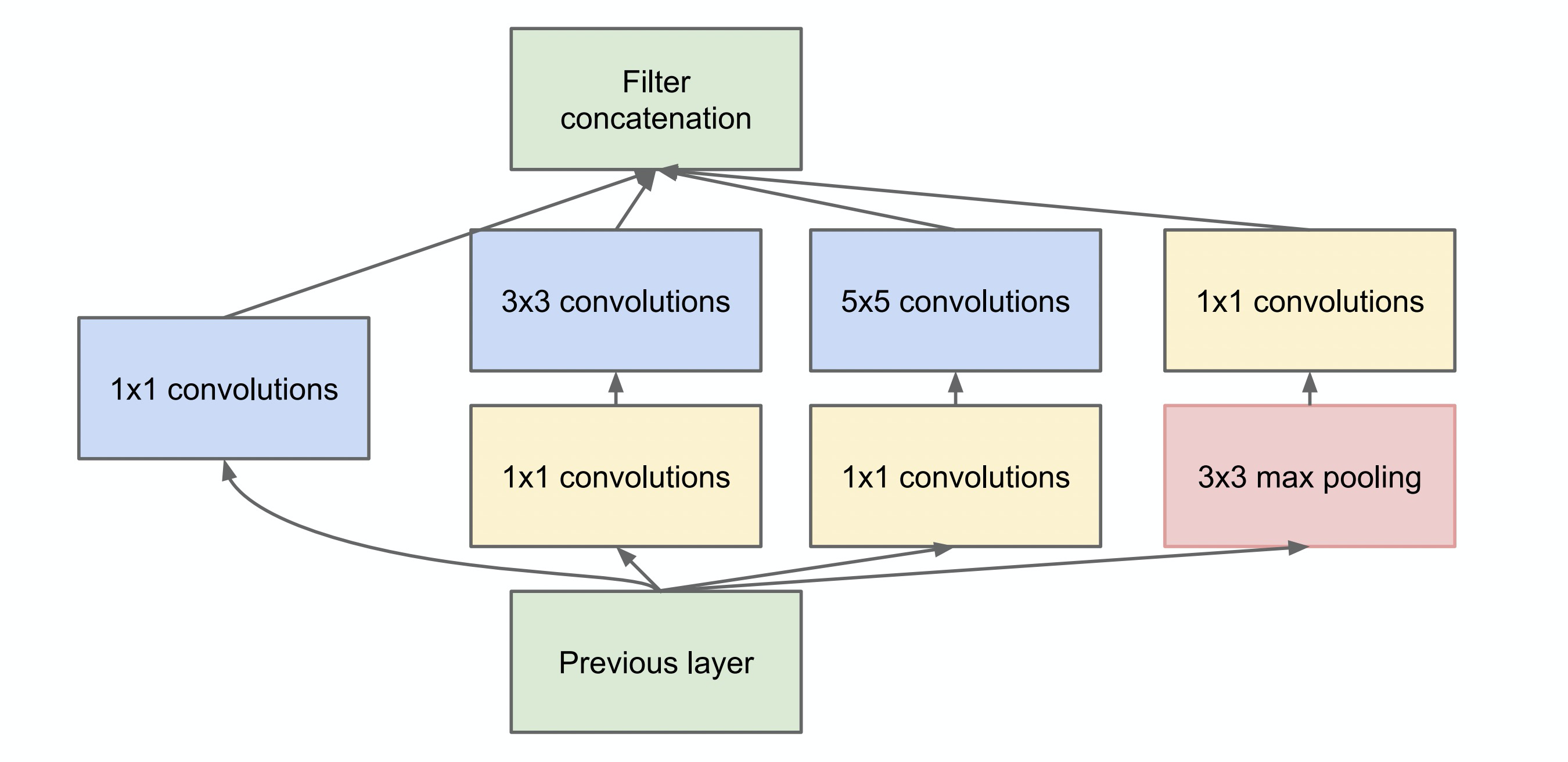GooLeNet就是由以上的Inception模块构成的一个22层网络。别看网络层数有22层，但是它参数量却比AlexNet与VGG都要少，这带来的优势就是，搭建起来的模型就很小，占的存储空间也小。具体的网络结构你可以参考它的论文。

### ResNet

ResNet中文意思是残差神经网络。在2015年的ImageNet比赛中，模型的分类能力首次超越人眼，1000类图片top-5的错误率降低到3.57%。

#### 网络退化问题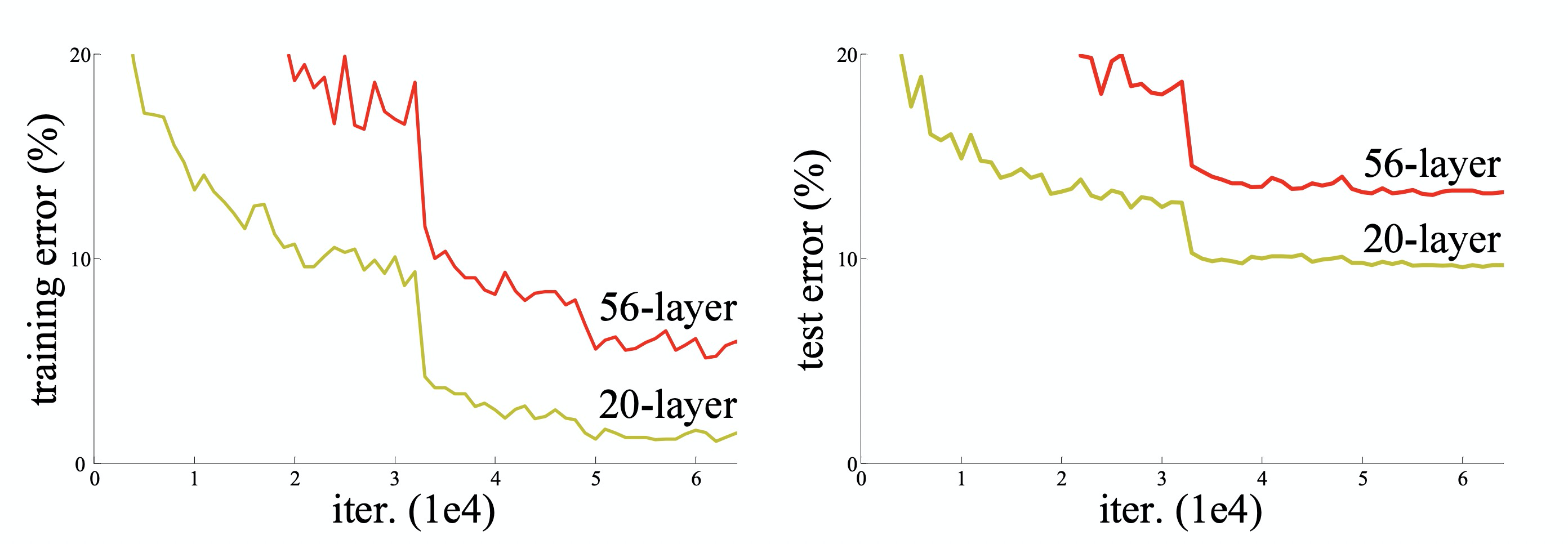#### 残差学习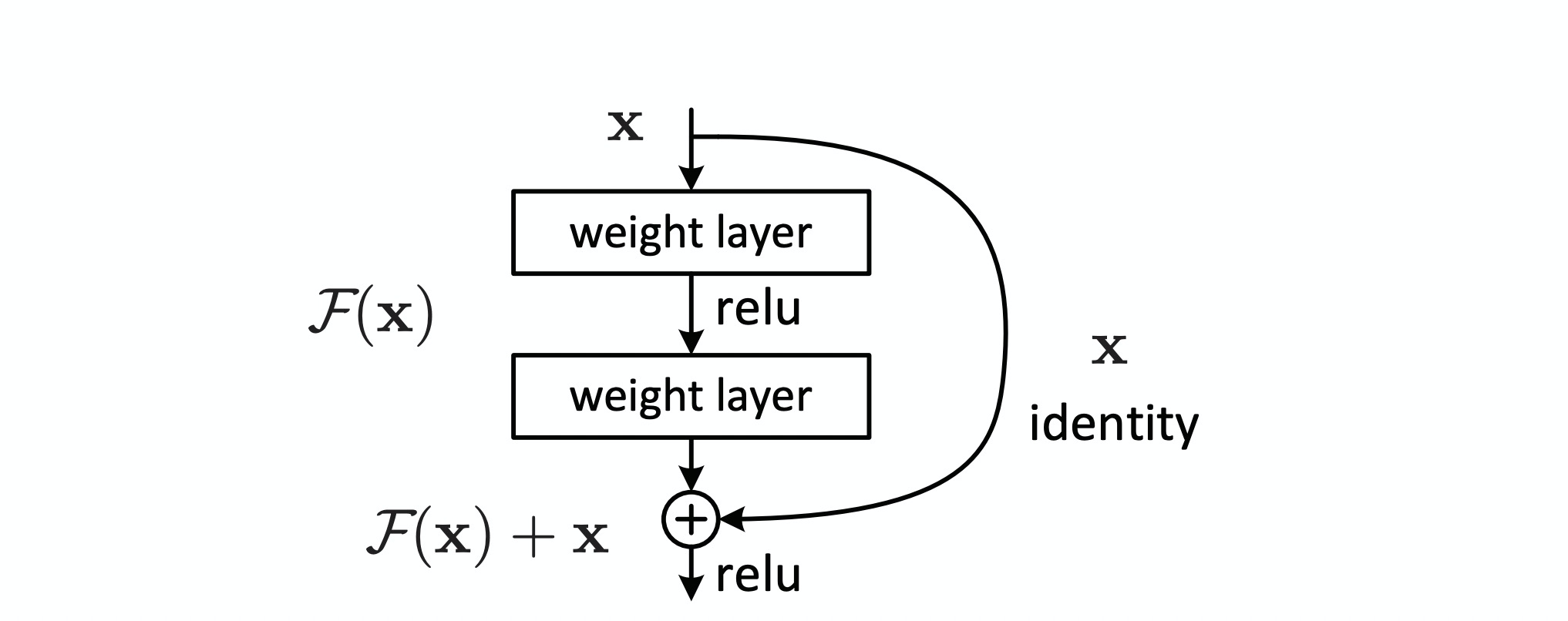$y = F(x, W\_i) + x$

F可以是2层的卷积层。也可以是3层的卷积层。最后作者发现，通过残差块，就可以训练出更深、更加优秀的卷积神经网络了。

## 小结